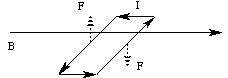# Mass spectroscopy, Current Loops and Spin

Consider a charge q moving with velocity v in a magnetic field. Since q v has the same units as I L, the moving charge acts like a current; the resulting magnetic force is called the "Lorentz Force":

F = q v B sin q,

where q is the angle between v and B. The direction of the force is perpendicular to both B and v.

Recall that in uniform circular motion, the centripetal force (which keeps the object moving in a circle) is perpendicular to the velocity. Assume that we have a constant B field which is perpendicular to the plane of motion of a charged particle. The resultant force will be in the plane, but perpendicular to the velocity, so the particle will travel in a circle. Equating the Lorentz Force to centripetal force,we have

q v B = m v 2 / r,

or

m = r q B / v.

This gives us a way to measure the mass of an ion of known charge and velocity. The radius of the circle allows us to compute the mass of the ion. This measurement technique is called "mass spectroscopy".

Now consider a current loop in the shape of a rectangle (the shape is actually not important) of length l and width w:In a (constant) magnetic field, the current elements along the long sides will experience forces (drawn with dashed arrows above). The forces experienced by the end current elements are initially zero, but as the loop moves, the forces will attempt to lengthen the loop; we will assume the loop is rigid and ignore those forces. The net forces have a magnitude of I l B, and cause a torque with lever arm w / 2:

t = I l B w / 2 + I l B w / 2

= I A B,

where A is the area of the rectangle. In general, we write the torque as

t = m B sin q.

Here q is the angle between B and the "normal" to the surface bounded by the loop, and

m = n I A

is called the "magnetic moment" of the loop: n is the number of turns in the loop, I is the current, and A is the surface area of the (planar) shape circumscribed by the loop. The direction of the magnetic moment (the normal to the loop) is found by another right hand rule:

3. Curl your fingers (of your right hand!) around the current flowing through the loop; your thumb will point in the direction of the magnetic moment.

The torque rotates the loop to line the magnetic moment up parallel to B. The energy of the loop in the magnetic field is

U = - m B cos q,

and we see that the process of aligning the moment with the external magnetic field B minimizes the potential energy of the loop: as q goes to 0, U reaches a minimum.

Finally, consider a rotating charged conducting ball (so that all of the excess charge is on the surface). If you slice the ball up perpendicularly to its axis of rotation into very small cylinders, each cylinder will behave as a current loop. This means that a rotating charged ball has a magnetic moment. While it is virtually certain that electrons and protons are NOT rotating conducting charged balls, we do model them as spinning charges and therefore they too have a magnetic moment. Their angular momentum is called "spin". This spin is quantized in units of h ("Planck's Constant" = 6.626 x 10 - 34 J s; note that these units are units of angular momentum). It happens that in a magnetic field, an electron or a proton can only have spins of h / 2 or - h / 2 ("up" or "down" with respect to the direction of the field). Why this should be is another of the mysteries of physics.

The next section is about Magnetic Resonance Imaging.

If you have stumbled on this page, and the equations look funny (or you just want to know where you are!), see the College Physics for Students of Biology and Chemistry home page.